# Kindergarten Addition Worksheets

## Free addition worksheets for preschool and kindergarten

These free worksheets focus on basic addition skills.  The emphasis is on addition using pictures as a visual aid to help build an understanding of the concept of addition.  We also use visual manipulatives in our online math lessons for K-5 students.

## Count the objects and add the numbers (up to 10)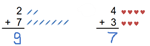Each of these count and add worksheets represents each number in the addition equation with objects to emphasize that addition can be performed by counting up.

## Count the objects, write the equation and solve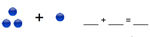These addition with pictures worksheets represent the addition question first with pictures, asking students to write the equation by counting and filling in the blanks.

## Draw more circles to make 10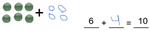These making 10 with objects worksheets provide practice in making 10, a critical skill in numeracy.

## Add the objects (up to 20)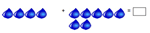These addition with pictures worksheets have sums up to 20.

## Make up numbers in 3 different ways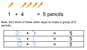Each of these making numbers worksheets ask students to make a number (4-10) in 3 different ways.

## Add the numbers together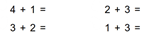These addition worksheets give practice in adding single digit numbers.  Separate worksheets for sums to 5, sums to 10 and sums to 20.

## Add these numbers together (vertical)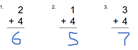These vertical addition worksheets give practice in adding single digit numbers in vertical form.  Separate worksheets for sums up to 10 and sums up to 20.

## Fill in the missing number to make 10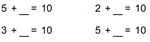Our making 10  worksheets ask students to find the missing number to make 10.  Fluency in this topic is a must!

## Fill in the missing number to make the "teen" number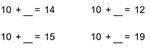These teen number worksheets emphasize the relationship between 10 and numbers 11-19.

## Read and solve these addition word problems.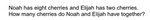Our kindergarten addition word problems worksheets provide simple addition problems, helping to connect addition to the real world.

## More addition worksheets

Find all of our addition worksheets, from adding by counting objects  to addition of multiple large numbers in columns.

## What is K5?

K5 Learning offers reading and math worksheets, workbooks and an online reading and math program for kids in kindergarten to grade 5.  We help your children build good study habits and excel in school.